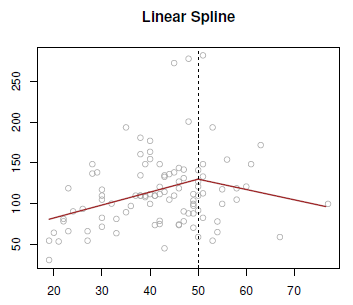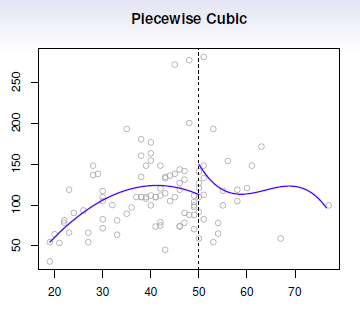# Data Mining - Step Function (piecewise constants)

Step functions, are another way of fitting non-linearities. (especially popular in epidemiology and biostatistics)

## Procedure

Continuous variable are cut into discrete sub-ranges and fit a constant model in each of the regions. It's a piecewise constant (model|function).

## Characteristics

### Natural cut point

The piecewise constant functions are especially useful if they are some natural cut points that you want to use or/and are of interest.

### Summary

what is the average income for somebody below the age of 35? You read it straight off the plot. This is often good food for summaries (in newspapers, reports, …)

### Interaction

Useful way of creating interactions that are easy to interpret.

Example of interaction variable (between Year and Age) in a linear model:

X_1 = I(Year < 2005) . Age
X_2 = I(Year > 2005) . Age


where I is the R indicator function.

With this two variables, we will fit a different linear model as a function of age for the people who worked before 2005 and those after 2005. We will get two different linear functions in each age category. It's an easy way of seeing the effect of an interaction.

### Local

One advantage over polynomial is that the calculation is local.

With polynomials, we have a single function for the whole range of the x variable. If I change a point on the left side, it could potentially change the fit on the right side for polynomials. But for step functions, a point only affects the fit in its partition and not the others.

### Knots

Choice of cutpoints or knots can be problematic. For creating non-linearities, smoother alternatives such as splines are available.

## Implementation

The function of the sub-ranges must be seen as binary variable.

Is x less than 35?

• If yes, you make it 1.
• If not, you make it a 0.

You creates then a series of dummy variables (zero-one variables) representing each group and you just fit those with the linear model.

## Models

In the below plot, there is a cut:

• at 35
• at 50 (not really visible in the regression plot)
• and at 65.

$$C_1(X) = I(X < 35); C_2(X) = I(35 \leq X < 50), \dots, C_3(X) = I(X \geq 65)$$

### Logistic

Discover MoreStatistics - (Linear spline|Piecewise linear function)

A linear spline, or piecewise linear function has a degree zero and is: linear in the left and the right. forced to be continuous at the knot. Just like the global polynomials and the piecewise...Statistics - Non-linear (effect|function|model)

Non-linear effect The truth is almost never linear but often the linearity assumption is good enough. (Linearity is an approximation) When its not (increasing in complexity): polynomials, step functions,...Statistics - Piecewise polynomials

Piecewise polynomials generalize the idea of piecewise constants. The idea try to get rid of the global polynomial because it's global and not local. Instead of having asingle polynomial over the whole...